Students can cross-reference their work with ICSE Class 9 Maths Solutions S Chand Chapter 11 Rectilinear Figures Ex 11(A) to ensure accuracy.

## S Chand Class 9 ICSE Maths Solutions Chapter 11 Rectilinear Figures Ex 11(A)

Question 1.
Calculate the angles marked with small letters in the diagrams drawn in the figures.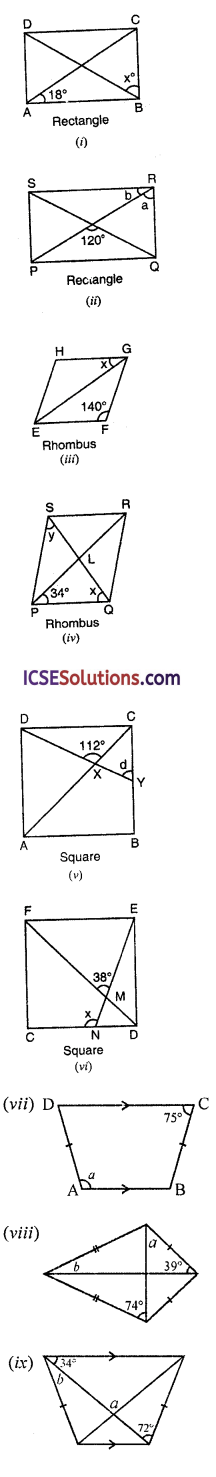Solution:
(i) ∵ In a rectangle, diagonals are equal and bisect each other at O
∴ AO = BO
∴ ∠OAB = ∠OBA = 18° (given)
But ∠ABC = 90° (Angles of a rectangle)
∴ x = 90° – 18° = 72°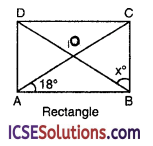(ii) In rectangle PQR.S, its diagonals bisects each other at T
∠PTQ = 120°.
∴ ∠TPQ + ∠TQP = 180°- 120° = 60°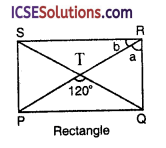But PT = QT
∴ ∠TPQ = ∠TQP = $$\frac { 60 }{ 2 }$$ = 30°
Now ∠SRT = ∠TPQ (alternate angles)
∴ b = 30°
But a + b = 90° (angles of a rectangle)
⇒ a + 30° = 90° ⇒ a = 90° – 30° = 60°
∴ a = 60°, b = 30°

(iii) In the rhombus EFGH,
∠EFG = 140°
But ∠EFG + ∠FGH = 180° (co-linear angles)
⇒ 140° +∠FGH = 180°
⇒ ∠FGH = 180°- 140° = 40°
∵ Diagonals of a rhombus bisects the angles
∴ x = $$\frac { 1 }{ 2 }$$ x 40° = 20°

(iv) ∵ The diagonaLs of a rhombus bisect each-other at right angles
∴ ∠PLQ = 90°
Now in ∆PLQ
∴ ∠LPQ + ∠LQP = 90°
⇒ 34° + x = 90° ⇒ x = 90° – 34°
x = 560
∵ Diagonal QS bisects ∠Q and ∠S
∴ x = y ⇒ y = 56°
Hence x = 56°, y = 560

(v) In the square ABCD,
Diagonal AC intersects a line DY such that
DY such that
∠DXC = 112°
∵ Diagonal AC bisects the angles A and C
∴ ∠ACB on ∠XCY = 45°
But ∠CXY + ∠CXD = 180°
(Linear pair)
∴ ∠CXY + 112°= 180°
⇒ ∠CXY = 180° – 112° = 68°
Now in ∆CXY,
∠CXY + ∠XYC + ∠XCY = 180°
⇒ 68° + d + 45° = 180°
⇒ 113° + d = 180°
⇒ d = 180° – 113°
⇒ d = 67°
∴ d = 67°(vi) In square CDEF,
DF is its diagonal
EN is a line segment which intersects DF at M such that ∠EMF = 38°, ∠MNC = x
∵ ∠EMF = ∠DMN
(vertically opposite angles)
∴ DMN = 38°
But diagonal DF bisects the ∠D and ∠F
∴ ∠MDN = 45°
In ∆MND,
Ext. ∠MNC = ∠DMN + ∠MDN
⇒ x = 38° + 45° = 83°
Hence x= 83°

(vii) In the figure,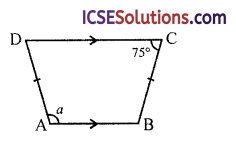AB || DC and AD = BC
∠A = a,∠C = 75°
∵ ABCD is an isosceles trapezium
∴ ∠A + ∠C = 180° and ∠B + ∠D = 180°
Now∠A + ∠C = 180°
⇒ a + 75° = 180° ⇒ a = 180° – 75°
∴ a = 105°

(viii) The figure is of a kite whose diagonals AC and BD are perpendicular to each other.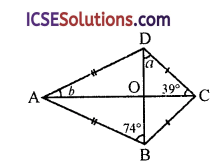AB = AD and CB = CD
Now, in right ∠OCD, ∠DOC = 90°
∴ a + 39° = 90° ⇒ a = 90° – 39° = 51°
∴ ∠ADO = ∠ABO = 90°
and in right ∆AOD, ∠AOD = 90°
∴ b + ∠ADO = 90°
⇒ b + 74° = 90°
∴ b = 90° – 74° = 16°

(ix) ABCD is an isosceles trapezium in which AB || DC
AC and BD intersect each other at O
∠ODC = ∠OCD = 34°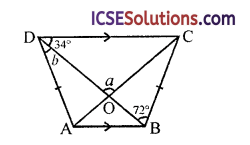But in ∆OCD,
a + ∠ODC + ∠OCD = 180°
a + 34° + 34° = 180° ⇒ a + 68° = 180°
∴ a = 180° – 68° = 112°
and ∠ADB = ∠ACB = b
∴ ∆OBC
Ext. a = 12° + b
⇒ 112° = 72 ° + b
⇒ b = 112° – 72° = 40°
Hence ∠A = 112° and ∠B = 40°

Question 2.
Find each angle of a parallelogram if two consecutive angles are in the ratio of 1 : 5.
Solution:
In parallelogram ABCD,
∠A : ∠B = 1 : 5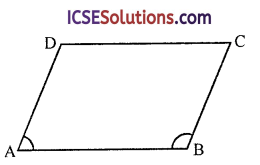Let ∠A = x, then ∠B = 5x
But ∠A + ∠B = 180° (co-interior angles)
∴ x + 5x – 180° ⇒ 6x = 180°
⇒ x = $$\frac { 180 }{ 6 }$$ = 30°
∴ ∠A = 30°, ∠B = 5x = 5 x 30° = 150°
and ∠C = ∠A = 30 and ∠D = ∠B = 150°

Question 3.
Find the size of the angles of a parallelogram if one angle is 20 less than twice the smallest angle.
Solution:
Let the smallest angle = x
Then other greatest angles = 2x – 20
But x + 2x – 20 = 180°(co-interior angles)
3x – 20 = 180 ⇒ 3x = 180 + 20 = 200
∴ x = $$\frac { 200 }{ 3 }$$ = 66$$\frac { 2 }{ 3 }$$
∴ Smallest angle = 66$$\frac { 2° }{ 3 }$$
and greater angle = 2x – 20
= 2 x $$\frac { 200 }{ 3 }$$ – 20
= $$\frac { 400 }{ 3 }$$ – 20 = $$\frac { 400-60 }{ 3 }$$
= $$\frac { 340 }{ 3 }$$ = 113 $$\frac { 1° }{ 3 }$$
∴ The angles of parallelogram are
$$66 \frac{2^{\circ}}{3}, 113 \frac{1^{\circ}}{3}, 66 \frac{2^{\circ}}{3} \text { and } 113 \frac{1^{\circ}}{3}$$

Question 4.
If an angle of rhombus is 50°, find the size of the angles of one of the triangles which are formed by the diagonals.
Solution:
ABCD is a rhombus in which ∠A = 50° and its diagonals AC and BD bisect each-other at O right angles∴ ∠AOB = 90°
∵ Diagonals bisect the opposite angles 50°
∠OAB = $$\frac { 50° }{ 2 }$$ = 25°
∠OBA = 180° = (90° + 25°)
= 180°- 115° = 65°
Hence angles are 25°, 65° and 90°.Question 5.
The diagonals AC and BD of a rectangle ABCD intersect eachother at P. If ∠ABD = 50°, find ∠DPC.
Solution:
In rectangle ABCD, diagonals AC and BD bisect eachother at P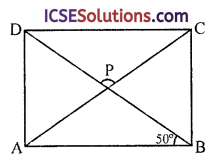∠ABD = 50°
In ∆APB,
AP = BP
(∵ The diagonals bisect eachother)
∴ ∠PAB = ∠PBA or ∠ABD = 50°
∴ ∠APB = 180° – (50 + 50°)
= 180°- 100° = 80°
But ∠CPD = ∠APB
(vertically opposite angles)
∴ ∠CPD = 80°

Question 6.
If an angle of parallelogram is two-third its adjacent angle, find the angles of the parallelogram.
Solution:
In parallelogram ABCD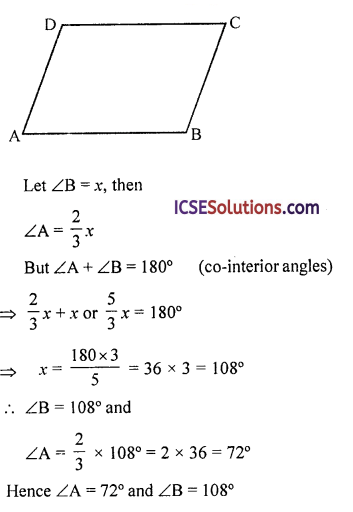Question 7.
In the figure, ABCD is a parallelogram in which ∠DAB = 70°, ∠DBC = 80°. Calculate angles CDB and ADB.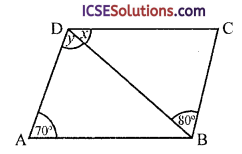Solution:
In parallelogram ABCD,
BD is its diagonal
∠DAB = 70°, ∠DBC = 80°
∠CDB = x and ∠ADB = y
∵ AD || BC and BD is its transversal
⇒ y = 80°
In ∆ABD,
∠DAB + ∠ABD + ∠ADB = 180°
(sum of angles of a triangle)
⇒ 70° + ∠ABD + 80°= 180°
⇒ 150° + ∠ABD = 180°
⇒ ∠ABD = 180° – 150° = 30°
But ∠CDB = ∠ABD (alternate angles)
⇒ x = 30°
Hence ∠ABD = 30° and ∠ADB = 80°

Question 8.
Calculate the side of a rhombus if its diagonals are 18 cm and 24 cm respectively.
Solution:
In a rhombus ABCD, diagonals AC = 24 cm, and BD = 18 cm∵ The diagonals of a rhombus bisect each other at right angles
∴ AO = OC = $$\frac { 24 }{ 2 }$$ = 12 cm
BO = OD = $$\frac { 18 }{ 2 }$$ = 9 cm
and ∠AOB = 90°
∴ In right ∆AOB
AB² = AO² + BO² (Pythagoras Theorem)
= (12)² + (9)²
= 144 + 81 = 225
= (15)²
∴ AB = 15
∴ Each side of rhombus ABCD = 15 cm

Question 9.
The sides of a rhombus are 5 cm and one diagonal is 8 cm. Calculate (i) the length of the other diagonal and (ii) the area of the rhombus. Solution:
In rhombus ABCD,
AC and BD are its diagonals AB = 5 cm and AC = 8 cm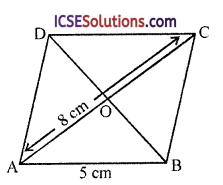∵ The diagonals of a rhombus bisect each other at right angles
∴ AO = OC = $$\frac { 8 }{ 2 }$$ = 4 cm and BO = OD and
∠AOB = 90°
Now in right ∆AOB
AB² = AO² + BO² (Pythagoras Theorem)
⇒ (5)² = (4)² + BO²
⇒ 25 = 16 + BO²
⇒ BO² = 25 – 16 = 9
⇒ BO² = 9 = (3)²
∴ BO = 3
∴ BD = 2BO = 2 x 3 = 6 cm
Now area of rhombus
= $$\frac{\text { Product of diagonals }}{2}$$
$$\frac{\mathrm{AC} \times \mathrm{BD}}{2}=\frac{8 \times 6}{2}$$ = 24 cm²Question 10.
PQRS is a rhombus :
(i) If it is given that PQ = 3 cm, calculate the perimeter of PQRS.
(ii) If the height of the rhombus is 2.5 cm, calculate the area.
(iii) If the diagonals cut at O, state the size of the angle POQ in degrees. (ICSE)
Solution:
In rhombus ABCD
Side PQ = 3 cm
Height RL = 2.5 cm
and diagonals AC and BD cut each other at OTo find :
(i) Perimeter of PQRS
(ii) Area of PQRS
(iii) Measure of ∠POQ
(i) Perimeter = 4 x side
= 4 x 3 cm = 12 cm

(ii) Base PQ = 3 cm and height RL = 2.5 cm
∴ Area of PQRS = Base x Height
= 3 x 2.5 = 7.5 cm²

(iii) The diagonals of a rhombus bisect each- other at right angles
∴ ∠POQ = 90°

Question 11.
ABCD is a trapezium with AB parallel to DC. If AB = 10 cm, AD = BC = 4 cm and ∠DAB = ∠CBA = 60°, calculate
(i) the length of CD;
(ii) the distance between AB and CD.
Solution:
ABCD is a trapezium in which AB = 10 cm, AD = 4 cm
∠DAB = ∠CBA = 60°Draw CL ⊥ AB and DM ⊥ AB
∵ ∠DAB = ∠CBA (each = 90°)
∴ ABCD is an isosceles trapezium
AD = BC = 4 cmHence
(i) CD = 6 cm
(ii) Distance between AB and CD = 2$$\sqrt{3}$$ cm

Question 12.
Find the value of y so that EFGH is isosceles.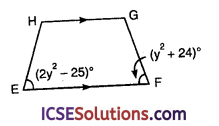Solution:
EFGH is an isosceles trapezium
∴ EH = FG
and ∠HEF = ∠GFE
⇒ 2y² – 25 = y² + 24°
2y² – y² = 24 + 25
⇒ y² = 49 = (±7)²
∴ y = 7 or – 7

Question 13.
PQRS is a rhombus in which the diagonal PR is produced to T. If ∠SRT = 152°, then find x, y and z.Solution:
In rhombus PQRS, PR and QS are the diagonals which intersect each other at O
PR is produced to T
∠SRT = 152°
∵ ∠PRS + ∠SRT = 180° (Linear pair)
⇒ ∠PRS + 152°= 180°
⇒ ∠PRS = 180°- 152° = 28°
∴ ∠SPQ = ∠SRQ = 2∠PRS
= 2 x 28° = 56°
(∵ Diagonals of a rhombus bisect the angles)
But ∠PQR + ∠SRQ = 180°
(co-interior angles)
⇒ ∠PQR + 56°= 180°
⇒ ∠PQR = 180° – 56° = 124°
∴ x = $$\frac { 1 }{ 2 }$$∠PQR = $$\frac { 1 }{ 2 }$$ x 124° = 62°
∴ x = 62°
y = 90°
(∵ Diagonals of rhombus bisect each other at right angles)
z = ∠PRS = 28°

Question 14.
In the figure, ABCD is a rhombus and ABE is an equilateral triangle ∠BCD = 78°. Calculate ∠ADE, ∠BDE and ∠BED.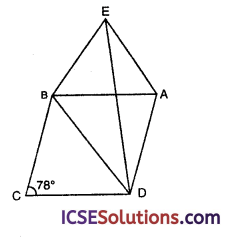Solution:
ABCD is a rhombus and ∆ABE is an equilateral triangle
BD and DE are joined ∠BCD = 78°
and ∠EAB = 60°
(angle of equilateral triangle)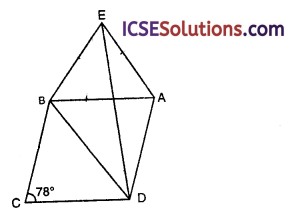∠EAD = 78° + 60° = 138°
But ∠AED + ∠ADE + ∠EAD = 180° (angles of a triangle)
⇒ 2∠ADE = 180° – 138° = 42°
(i) ∴ ∠ADE = $$\frac { 42 }{ 2 }$$ = 21°

(ii) ∠AED = 60°
∴ ∠BED = ∠AEB – ∠AED = 60° – 21° = 39°

(iii) ∠BCD + ∠CDA = 180° (co-interior angles)
∴ ∠CDA = 180° – ∠BCD = 180° – 78° = 102°
BD is the diagonal of rhombus
∴ ∠BDA = $$\frac { 1 }{ 2 }$$ ∠CDA = $$\frac { 1 }{ 2 }$$ x 102° = 51°
∴ ∠BDE = 51° – 21° = 30°
Hence ∠ADE = 21°, ∠BDE = 30° and ∠BED = 39°

Question 15.
In the figure, equilateral AEBC surmounts square ABCD. Find ∠BED represented by x.Solution:
ABCD is a square and EBC is an equilateral triangle on BC
ED is joined
In ∆ECD,
CD = CE (given)
∠CED = ∠CDE
∴ (angles opposite to equal sides)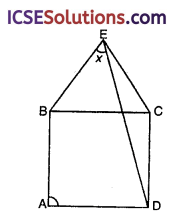But ∠DCE = ∠DCB + ∠BCE
= 90° + 60° = 150°
∴ ∠CED + ∠CDE = 180° – 150° = 30°
⇒ ∠CED + ∠CED = 30°
⇒ 2∠CED = 30° ⇒ ∠CED = $$\frac { 30° }{ 2 }$$ = 15°
But ∠BEC = 60°
(angle of an equilateral triangle)
∴ ∠BED = ∠BEC – ∠CED = 60°- 15° = 45°

Question 16.
ABCD is a square. ABO is an equilateral triangle inside the square. Find ∠DOC.
Solution:
ABCD is a square
∴ Its each angle = 90°
ABO is an equilateral triangle∴ ∠AOD = ∠ADO = $$\frac { 150° }{ 2 }$$ = 75°